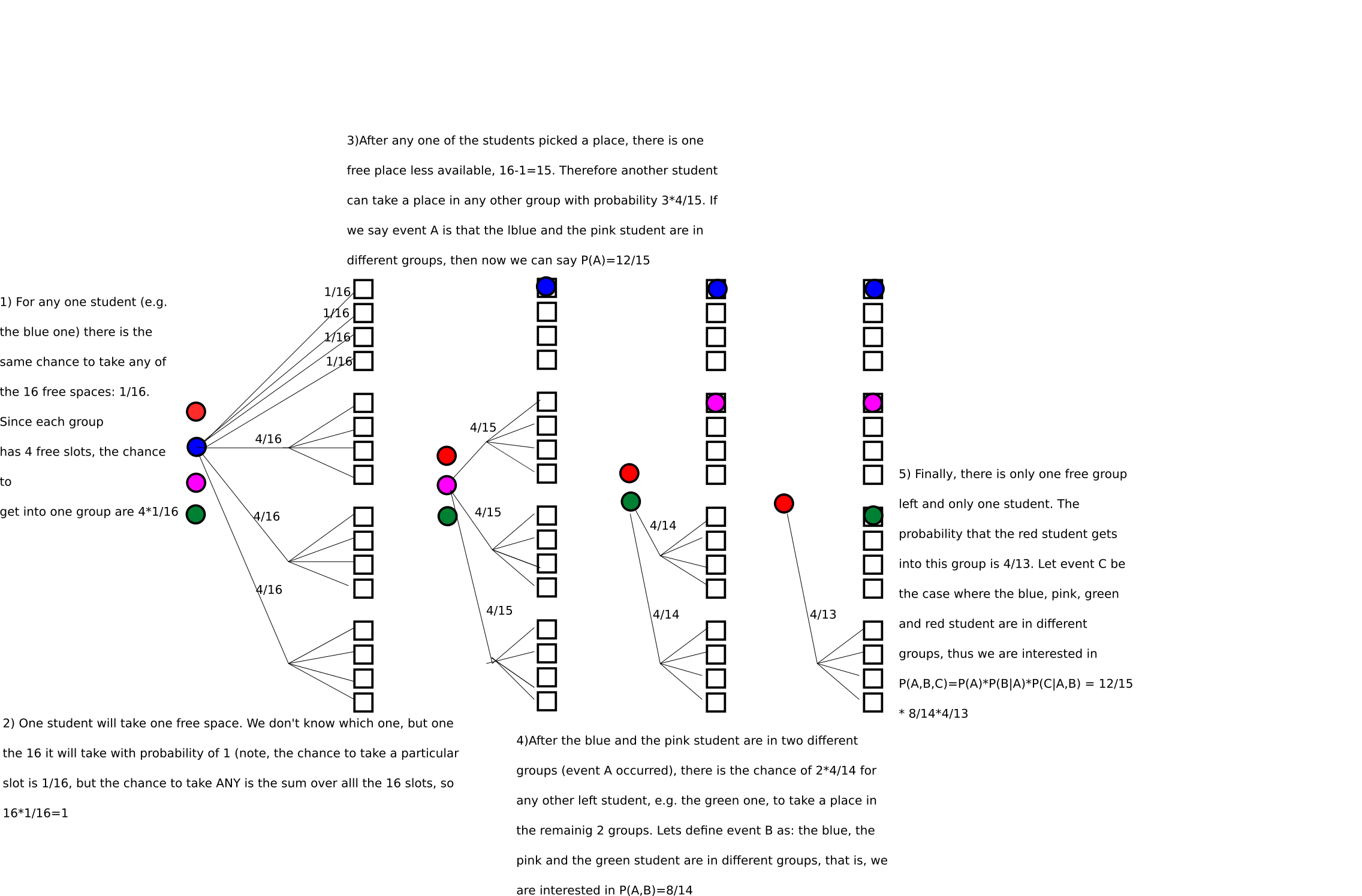# Counting

Herleitung der Formeln für Kombin etc.

There is a thing I learned. When you draw a probability tree, I often assumed that all the outcomes are equally likely. Then I would count the outcomes that were in my favor (belonging to some event A) and put it into relation to the number of events  that were possible in total, i.e. using the naive definition of probability. But that doesent always work. Give the example here, where I did the 5 white balls nad 3 blacks one drawing with replacement. I used the formula. If you use the formula below for counting the number of combinations where order does not count but with replacement, you get a number, but the probabilities are not the same for each occurrence anymore.

\binom{n+k-1}{k}

which gives the amount of possibilities for combinations (order does not matter) with replacement